Updating search results...

# 9 Results

View
Selected filters:
• MCCRS.Math.Content.3.MD.C.7d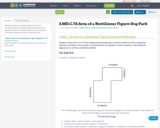Conditional Remix & Share Permitted
CC BY-NC-SA
Rating
0.0 stars

This task requires students to find the area of a rectilinear figure and model their thinking through multiple equations. This task relates to the Common Core State Standards: 3.MD.C.7d and 3.OA.D.8.

Subject:
Education
Mathematics
Material Type:
Activity/Lab
Assessment
Homework/Assignment
09/17/2018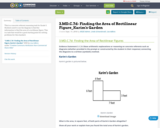Conditional Remix & Share Permitted
CC BY-NC-SA
Rating
0.0 stars

This is a concrete referent reasoning task for Grade 3. Students need to use the diagram to find the information to find the area of a rectilinear figure. This is a task that would be a good starting point for solving problems for this standard.

Subject:
Education
Elementary Education
Mathematics
Material Type:
Activity/Lab
09/17/2018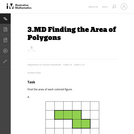Unrestricted Use
CC BY
Rating
0.0 stars

This is a task from the Illustrative Mathematics website that is one part of a complete illustration of the standard to which it is aligned. Each task has at least one solution and some commentary that addresses important asects of the task and its potential use. Here are the first few lines of the commentary for this task: Find the area of each colored figure. Each grid square is 1 inch long....

Subject:
Mathematics
Material Type:
Activity/Lab
Provider:
Illustrative Mathematics
Provider Set:
Illustrative Mathematics
Author:
Illustrative Mathematics
05/27/2013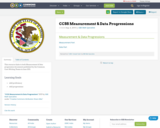Conditional Remix & Share Permitted
CC BY-SA
Rating
0.0 stars

This resource links to both Measurement & Data progression documents published by the Common Core Writing Teams in June 2011.

Material Type:
Assessment
Homework/Assignment
Teaching/Learning Strategy
08/08/2019Unrestricted Use
CC BY
Rating
0.0 stars

Subject:
Mathematics
Material Type:
Assessment
Homework/Assignment
Lesson Plan
01/28/2016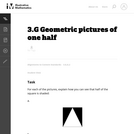Unrestricted Use
CC BY
Rating
0.0 stars

This task presents students with some creative geometric ways to represent the fraction one half. The goal is both to appeal to students' visual intuition while also providing a hands on activity to decide whether or not two areas are equal.

Subject:
Geometry
Mathematics
Material Type:
Activity/Lab
Provider:
Illustrative Mathematics
Provider Set:
Illustrative Mathematics
Author:
Illustrative Mathematics
10/01/2012Unrestricted Use
CC BY
Rating
0.0 stars

This is a rectangle subdivision task; ideally instead of counting each square. students should break the letters into rectangles, multiply to find the areas, and add up the areas. However, students should not be discouraged from using individual counting to start if they are stuck. Often students will get tired of counting and devise the shortcut method themselves.

Subject:
Mathematics
Measurement and Data
Material Type:
Activity/Lab
Provider:
Illustrative Mathematics
Provider Set:
Illustrative Mathematics
Author:
Illustrative Mathematics